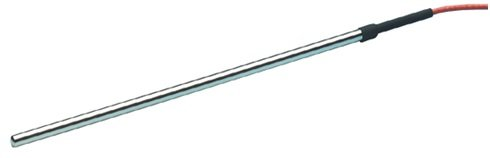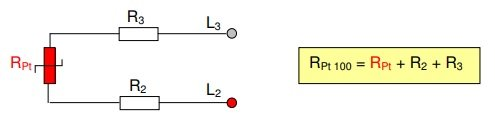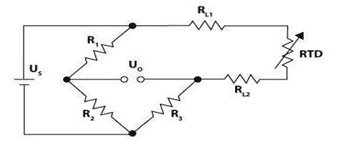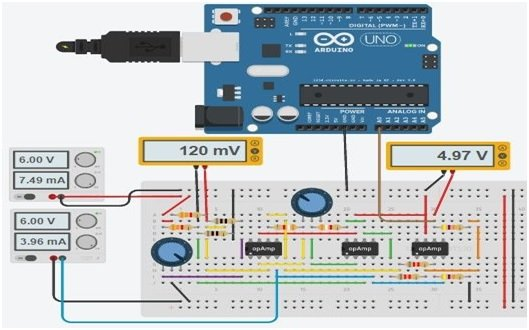# PT100 RTD Sensor introduction working and pinout

What is PT100 RTD Sensor? This (resistance temperature detector) sensor is a such type of sensor whose resistance is changed when  surrounding temperature is changed. It measures the temperature with the help of a resistance. The value of this resistance is directly proportional to temperature. It is constructed  in the form of a prob which composed of an element and this element is made up with platinum, Nickle or copper material. Normally two standards are used for palatium RTD. First one is European standard which is also known as IEC or  DIN standard and second one is American standard.

The palatium wire which is used for RTD sensor has  electrical resistance 100 ohm  at 0C0 temperature and temperature co-efficient of 0.00385 O/O/°C. This PT100 RTD sensor has been using for various applications such as for measuring liquid temperature ,air and gas temperature and surface temperature etc. This type of sensor is easily available in market or online shop . A simple PT100 RTD sensor is shown in figure 1Figure 1 PT100 RTD sensor

### Pin Configuration of PT100 RTD Sensor

If we talk about wire then different types of PT100 RTD sensor consists of different wires such a two, three or four wire PT100 RTD sensor. Each type has different connection but here we shell only talk about two wire PT100 RTD sensor. Two wire  PT100 RTD sensor is shown in figure 1 with resistance formula.Figure 2 Two Wire PT100 RTD sensor

According to figure 2 the resistance values of R3 and R2  are included in measured values of  PT100 but these values could be cancelled during calibration. But the first problem of PT100 RTD sensor is that, how convert the change in resistance to change in voltage, so that the controller which interface with this sensor read this change. So that this work is done with the help of voltage divider circuit and for this purposes 80 ohm to 150 ohm resistances are used but this voltage divider circuit has dc off set which decrease the voltage dividing sensitivity during amplification.

### Working Principle of  PT100 RTD Sensor

This PT100 RTD sensor works on the principle of resistance change. Similarly ,when dc current is supplied to this sensor then this current changes the impedance of sensor resistor. When this impedance is changed then voltages are dropped, and these drop voltages are depending upon supply current and the nominal resistance of RTD resistor. During this whole process, heat is also produced which could be low by applying low current, normally 1mA current is used. RTD can connect in two ,three or four wire configuration but two wire configuration is simplest configuration. When it is connected in two wire configuration then it is connected in Wheatstone bridge circuit form for measuring output voltages shown in figure 3.Figure 3 Two Wire Configuration of RTD 100 in Wheatstone Bridge circuit

But this circuit has one disadvantage means when connecting leads are connected with this circuit then their resistance is added up in RTD resistance, so the exact error cannot calculate.

### How Interface PT100 RTD Sensor with Arduino Board?

For measuring temperature from RTD sensor, a controller is required for interfacing purposes and here we shell tell the user how interface the PT 100 RTD sensor with Arduino board. So, for interfacing purposes Wheatstone bridge is formed with help of three 741 operational amplifiers, two 3.9k ohm, two 3.3k ohm, two 8.2k ohm, two 47k ohm, one 5k ohm and one 80 ohm resistor. All these components are connected with two wire PT100 RTD sensor and then this whole circuit is interfaced with Arduino board according to figure 4Figure 4 Interfacing of RTD Sensor with Arduino Board

After making whole connection, a logic program is written in Arduino library according to whole connection then this logic program is up load in Arduino board with the help of Arduino IDE software. So, the temperature could be checked after running the logic program and changing the resistance of Wheatstone bridge. For different resistance temperature would be different.

Alternative Sensor Module